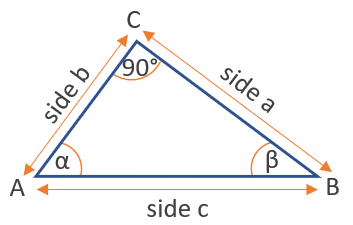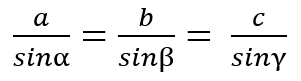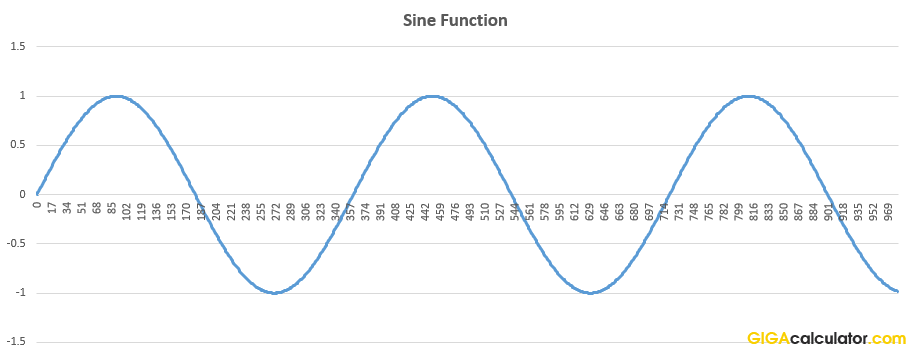# Sine Calculator

Use this sin calculator to easily calculate the sine of an angle given in degrees or radians. Calculating Sin(x) is useful in right triangles such as those formed by the heights in different geometric shapes.

### Calculation results

1 sin(90) =
Share calculator:

Embed this tool:
get code

## The Sine function ( sin(x) )

The sine is a trigonometric function of an angle, usually defined for acute angles within a right-angled triangle as the ratio of the length of the opposite side to the longest side of the triangle. In the illustration below, sin(α) = a/c and sin(β) = b/c.From cos(α) = a/c follows that the sine of any angle is always less than or equal to one. The function takes negative values for angles larger than 180°. Since for a right triangle the longest side is the hypotenuse and it is opposite to the right angle, the sine of a right angle is equal to the ratio of the hypotenuse to itself, thus equal to 1. You can use this sine calculator to verify this.

A commonly used law in trigonometry which is trivially derived from the sine definition is the law of sines:The sine function can be extended to any real value based on the length of a certain line segment in a unit circle (circle of radius one, centered at the origin (0,0) of a Cartesian coordinate system. Other definitions express sines as infinite series or as differential equations, meaning a sine can be an arbitrary positive or negative value, or a complex number.

## Related trigonometric functions

The reciprocal of sine is the cosecant: csc(x), sometimes written as cosec(x), which gives the ratio of the length of the hypotenuse to the length of the side opposite to the angle.

The inverse of the sine is the arcsine function: asin(x) or arcsin(x). The arcsine function is multivalued, e.g. arcsin(0) = 0 or π, or 2π, and so on. It is useful for finding an angle x when sin(x) is known.

## How to calculate the sine of an angle?

If the angle is known, then simply use our sine calculator which supports input in both degrees and radians. Once you have measured the angle, or looked up the plan or schematic, just input the measurement and press "calculate". This is how easy it is.

If the angle itself is unknown, one way to calculate the sine is to know the measurements of the lengths of the side opposite to it as well as the hypotenuse (side c in the figure). For example, if sin(α) is to be computed and the lengths of a and c are available, sin(α) = a / c. Other ways involve using the law of sines.

## Applications of the sine function

The sine function is usually used to model periodic phenomena in physics, biology, social sciences, etc. For example sound and light waves, day length and temperature variations over the year can be represented as a sine.Above: a wave generated using the sine function. A sine wave is the mirror image of a cosine wave.

Table of common sine values:

Common values of the sine function
0 0
30° π/6 0.50
45° π/4 0.707107
60° π/3 0.866025
90° π/2 1
120° 2π/3 0.866025
135° 3π/4 0.707107
150° 5π/6 0.50
180° π 0

#### Cite this calculator & page

If you'd like to cite this online calculator resource and information as provided on the page, you can use the following citation:
Georgiev G.Z., "Sine Calculator", [online] Available at: https://www.gigacalculator.com/calculators/sine-calculator.php URL [Accessed Date: 28 Sep, 2023].